Anúncio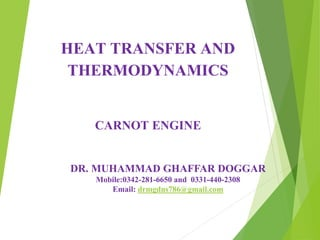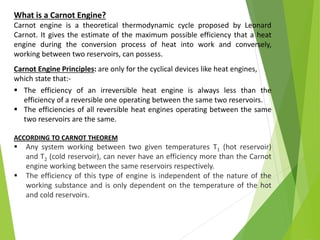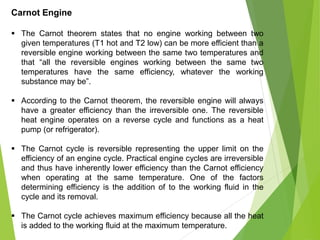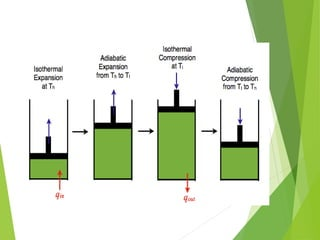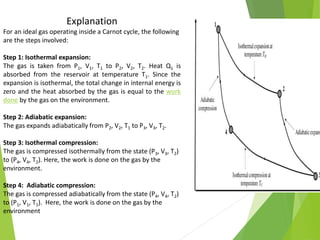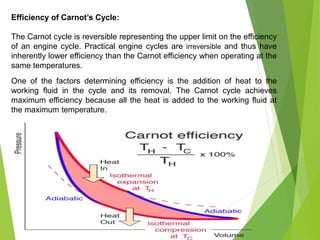1 de 8
Anúncio

### Lecture 5 Carnot enginepptx

1. DR. MUHAMMAD GHAFFAR DOGGAR Mobile:0342-281-6650 and 0331-440-2308 Email: drmgdns786@gmail.com CARNOT ENGINE HEAT TRANSFER AND THERMODYNAMICS
2. What is a Carnot Engine? Carnot engine is a theoretical thermodynamic cycle proposed by Leonard Carnot. It gives the estimate of the maximum possible efficiency that a heat engine during the conversion process of heat into work and conversely, working between two reservoirs, can possess. Carnot Engine Principles: are only for the cyclical devices like heat engines, which state that:-  The efficiency of an irreversible heat engine is always less than the efficiency of a reversible one operating between the same two reservoirs.  The efficiencies of all reversible heat engines operating between the same two reservoirs are the same. ACCORDING TO CARNOT THEOREM  Any system working between two given temperatures T1 (hot reservoir) and T2 (cold reservoir), can never have an efficiency more than the Carnot engine working between the same reservoirs respectively.  The efficiency of this type of engine is independent of the nature of the working substance and is only dependent on the temperature of the hot and cold reservoirs.
3. Carnot Engine  The Carnot theorem states that no engine working between two given temperatures (T1 hot and T2 low) can be more efficient than a reversible engine working between the same two temperatures and that “all the reversible engines working between the same two temperatures have the same efficiency, whatever the working substance may be”.  According to the Carnot theorem, the reversible engine will always have a greater efficiency than the irreversible one. The reversible heat engine operates on a reverse cycle and functions as a heat pump (or refrigerator).  The Carnot cycle is reversible representing the upper limit on the efficiency of an engine cycle. Practical engine cycles are irreversible and thus have inherently lower efficiency than the Carnot efficiency when operating at the same temperature. One of the factors determining efficiency is the addition of to the working fluid in the cycle and its removal.  The Carnot cycle achieves maximum efficiency because all the heat is added to the working fluid at the maximum temperature.
4. Explanation For an ideal gas operating inside a Carnot cycle, the following are the steps involved: Step 1: Isothermal expansion: The gas is taken from P1, V1, T1 to P2, V2, T2. Heat Q1 is absorbed from the reservoir at temperature T1. Since the expansion is isothermal, the total change in internal energy is zero and the heat absorbed by the gas is equal to the work done by the gas on the environment. Step 2: Adiabatic expansion: The gas expands adiabatically from P2, V2, T1 to P3, V3, T2. Step 3: Isothermal compression: The gas is compressed isothermally from the state (P3, V3, T2) to (P4, V4, T2). Here, the work is done on the gas by the environment. Step 4: Adiabatic compression: The gas is compressed adiabatically from the state (P4, V4, T2) to (P1, V1, T1). Here, the work is done on the gas by the environment
5. Efficiency of Carnot’s Cycle: The Carnot cycle is reversible representing the upper limit on the efficiency of an engine cycle. Practical engine cycles are irreversible and thus have inherently lower efficiency than the Carnot efficiency when operating at the same temperatures. One of the factors determining efficiency is the addition of heat to the working fluid in the cycle and its removal. The Carnot cycle achieves maximum efficiency because all the heat is added to the working fluid at the maximum temperature.
6. Video QUESTIONS AND SOLUTIONS
Anúncio